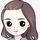cancel
Showing results for
Did you mean:Frequent Visitor

## Calendar, multiple dates but dates depend on each other

Goodmorning,

I am fairly new to Powerbi but loving it for the moment.

I have the following problem :

My data  :

Year PubYear Category Value.

2009  2015      x             100

2009   2019     x              105

2009  2019      y              200

2009  2021      x              110

2010  2015       x             120

2010  2021      x              125

2010 2021      y                200

and so on for over 1.4 million records.

The Client wants 2 visuals :

First year by year growth for the last pubyear per year

Second Year by Year growth voor 1 year over different pubyears

How can achieve this using a Calendartable or dont i have to use this table ?

1 ACCEPTED SOLUTIONCommunity Support

I created a sample pbix file(see the attachment), please check if that is what you want.

``````Year by year% =
VAR _selcat =
SELECTEDVALUE ( 'Table'[Category] )
VAR _selyear =
SELECTEDVALUE ( 'Table'[Year] )
VAR _selpubyear =
SELECTEDVALUE ( 'Table'[PubYear] )
VAR _prepubyear =
CALCULATE (
MAX ( 'Table'[PubYear] ),
FILTER (
ALLSELECTED ( 'Table' ),
'Table'[Category] = _selcat
&& 'Table'[Year] = _selyear
&& 'Table'[PubYear] < _selpubyear
)
)
VAR _prepyvalue =
CALCULATE (
SUM ( 'Table'[Value] ),
FILTER (
ALLSELECTED ( 'Table' ),
'Table'[Category] = _selcat
&& 'Table'[Year] = _selyear
&& 'Table'[PubYear] = _prepubyear
)
)
RETURN
IF (
ISBLANK ( _prepyvalue ),
BLANK (),
DIVIDE ( SUM ( 'Table'[Value] ) - _prepyvalue, _prepyvalue )
)``````In addition, you can refer the following videos to get it.

Power BI Year-Over-Year Growth % Calculations [DAX] - YouTube

Power BI YEAR over YEAR (YoY) Change Calculations [DAX]Best Regards

Community Support Team _ Rena
If this post helps, then please consider Accept it as the solution to help the other members find it more quickly.
2 REPLIES 2Community Support

I created a sample pbix file(see the attachment), please check if that is what you want.

``````Year by year% =
VAR _selcat =
SELECTEDVALUE ( 'Table'[Category] )
VAR _selyear =
SELECTEDVALUE ( 'Table'[Year] )
VAR _selpubyear =
SELECTEDVALUE ( 'Table'[PubYear] )
VAR _prepubyear =
CALCULATE (
MAX ( 'Table'[PubYear] ),
FILTER (
ALLSELECTED ( 'Table' ),
'Table'[Category] = _selcat
&& 'Table'[Year] = _selyear
&& 'Table'[PubYear] < _selpubyear
)
)
VAR _prepyvalue =
CALCULATE (
SUM ( 'Table'[Value] ),
FILTER (
ALLSELECTED ( 'Table' ),
'Table'[Category] = _selcat
&& 'Table'[Year] = _selyear
&& 'Table'[PubYear] = _prepubyear
)
)
RETURN
IF (
ISBLANK ( _prepyvalue ),
BLANK (),
DIVIDE ( SUM ( 'Table'[Value] ) - _prepyvalue, _prepyvalue )
)``````In addition, you can refer the following videos to get it.

Power BI Year-Over-Year Growth % Calculations [DAX] - YouTube

Power BI YEAR over YEAR (YoY) Change Calculations [DAX]Best Regards

Community Support Team _ Rena
If this post helps, then please consider Accept it as the solution to help the other members find it more quickly.Frequent Visitor

i tried you solution, but it is not what i wanted. I want to see the evolution through the years using their maximum publication year per categorie. So

For X 2009's max pubyear = 2021 for Y 2009's max pubyear = 2019, for 2010 for x and y it is 2021.

And on another visual i would want to see the evolution of 2009 through different pubyears.

So for X on 2009 i want to see 2015 vs 2019 vs 2021